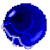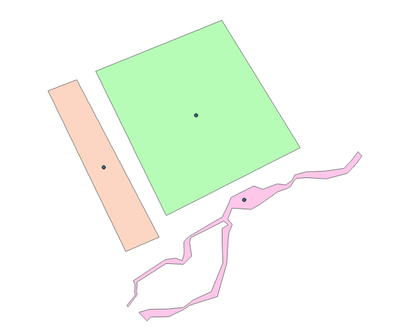# Cannot write multipart geometry parts with interior rings

773
5
06-05-2021 08:48 PM
Labels (1)by
New Contributor III

I am attempting to generate a center point for each part of a multipart geometry. The issue I am having is that when reading multipart geometries (some with holes), the internal rings are not retained when reading/writing the individual parts. For testing, I am copying each part to a memory feature class (see images where holes are ignored). Because of this, the "labelPoint" property is not working as I would like it to.

I am aware that I can use MultipartToSinglePart first, but I'd prefer to understand what part of this I'm not doing correctly, and handle the whole thing using Cursors.

``````# IMPORTS------------------------------------------------------------------#
import arcpy
import os
from arcpy.da import InsertCursor, SearchCursor

# INPUTS-------------------------------------------------------------------#
in_fc  = r'E:\SCRATCH.gdb\_MULTIPART_POLY'
out_fc = r'E:\SCRATCH.gdb\_CENTROID'

# MAIN---------------------------------------------------------------------#
# Create new output feature class.
arcpy.management.CreateFeatureclass(
out_path=os.path.dirname(out_fc),
out_name=os.path.basename(out_fc),
geometry_type='POINT',
template=in_fc,
spatial_reference=2248)

# Get relevant fields from the input FC to transfer. Add SHAPE@ token.
flds = [f.name for f in ap.ListFields(in_fc)
if f.type not in ['Geometry', 'OID']
and f.name not in ['Shape_Area', 'Shape_Length']]
flds.insert(0, 'SHAPE@')

# Read geometries, write centroids. Make TEMP copies of each geometry part.
with SearchCursor(in_fc, flds) as scurs, InsertCursor(out_fc, flds) as icurs:
for geom, *fields in scurs:
for i, part in enumerate(geom, start=1):

arcpy.management.CopyFeatures(arcpy.Polygon(part), fr'memory\Part{i}')
point_geom = getattr(arcpy.Polygon(part), 'labelPoint')

icurs.insertRow([point_geom, *fields])``````

The input polygon (one, multipart feature), followed by the output polygon parts and corresponding points:Input polygons.Separate output polygons, and corresponding label points.

Tags (3)
1 Solution

Accepted Solutionsby
New Contributor III

In case someone else has a similar issue in the future:

The solution to this was to nest the geometry "part" (which is already an Array) into another Array. Following this, the script works as expected. Full function below:

``````def FeatureToPoint(in_fc, out_fc, point_location='CENTROID', explode=False):
"""
Creates a new feature class of points from input polygons or polylines.

:point_location:
CENTROID- The true centroid if it is within or on the feature;
otherwise, the label point is returned.
TRUE_CENTROID- The center of gravity for a feature.
LABEL- The labelPoint is always located within or on a feature.
:explode: create center point for each part? or just each feature?
"""
import arcpy as ap
import os

from arcpy.da import SearchCursor, InsertCursor

crs = ap.da.Describe(in_fc)['spatialReference']

ap.management.CreateFeatureclass(
out_path=os.path.dirname(out_fc),
out_name=os.path.basename(out_fc),
geometry_type='POINT',
template=in_fc,
has_m='', has_z='',
spatial_reference=crs)

flds = [f.name for f in ap.ListFields(in_fc)
if f.type not in ['Geometry', 'OID']
and f.name not in ['Shape_Area', 'Shape_Length']]
flds.insert(0, 'SHAPE@')

center_type = {'CENTROID': 'centroid',
'TRUE_CENTROID': 'trueCentroid',
'LABEL': 'labelPoint',}

with SearchCursor(in_fc, flds) as scurs, InsertCursor(out_fc, flds) as icurs:
for geom, *fields in scurs:

if explode:
for i, part in enumerate(geom, start=1):

"""
'part' is already an Array. Not clear why it needs
to be nested into another Array, but it does. Otherwise, only
the exterior ring of the part gets converted to a polygon,
excluding any internal rings.
"""

exploded_polygon = ap.Polygon(ap.Array(part))

# Testing/visualizing outputs:
#ap.management.CopyFeatures(exploded_polygon, fr'memory\Part{i}')
point_geom = getattr(exploded_polygon, center_type[point_location])

icurs.insertRow([point_geom, *fields])

else:
point_geom = getattr(geom, center_type[point_location])
icurs.insertRow([point_geom, *fields])

return out_fc``````
5 RepliesbyMVP Esteemed Contributor

You have handled the case of reading a multipart to its individual parts, however, when it is read, only the exterior ring is read.  You need to provide logic to read the parts of the parts (ie the exterior and interior rings)

If a polygon contains holes, it consists of a number of rings. The array of point objects returned for a polygon contains the points for the exterior ring and all inner rings. The exterior ring is always returned first, followed by inner rings, with null point objects as the separator between rings. Whenever a script is reading coordinates for polygons in a geodatabase or shapefile, it should contain logic for handling inner rings if this information is required by the script; otherwise, only the exterior ring is read.

also note for polygons

centroid
The true centroid if it is within or on the feature; otherwise, the label point is returned.

I would compare your cursor approach to the MultipartToSinglepart tool  cursors offer little to no time advantage and the tool handles geometry cases well.

... sort of retired...by
New Contributor III

Thanks for the input Dan. I'm still a bit confused about this situation, however. I am (in theory, anyway) familiar with the way that ArcPy deals with geometry objects--the rings, and the Null points as separators.

When I add the following print statement, I am getting the exterior rings, followed by the interior rings, all separated by the null points:

(the first two lines are in the original post, and are just shown here for context)

``````    for geom, *fields in scurs:
for i, part in enumerate(geom, start=1):

print([array for array in part])   <---------LINE ADDED``````

I won't paste in what it prints (because it's long), but an example is basically:

[point, point, point, None, point, point, point, None, point, point, point]  --> which is exterior ring, interior ring, interior ring. This describes that middle polygon shown in the image with the two holes.

Based on this, the "part" variable contains all the rings that are necessary to create a polygon. So then why does my line below not create this polygon object, with these interior rings intact?:

``arcpy.management.CopyFeatures(arcpy.Polygon(part), fr'memory\Part{i}')``

You said, "when it is read, only the exterior ring is read." I don't follow what you mean here--if I was trying to get the .boundary() or something, that would make sense to me. But since the exterior and the interior rings are contained in the "part" object (evidenced by the print statement), why can't "part" be used to construct a Polygon object? The arcpy.Polygon() class doesn't read interior rings in this case? Would you be able to provide some code that would convert a polygon's part into a separate polygon?

In regard to the difference between 'centroid', 'trueCentroid', and 'labelPoint'--my code here is part of a larger script that allows the user to supply any of these. The above snippet is just an example to make it easier for folks to help me solve this issue. For now, the centroid is sort of a moot point, it's really the polygon situation that I would like to understand.

And as for MultipartToSinglepart--my goal is to learn about the inner workings of geometries, and why this isn't working the way I would expect it to. But you're right, I'll probably just revert to that once I (hopefully) learn why this approach is wrong.

Thanks again for all your help.by
New Contributor III

In case someone else has a similar issue in the future:

The solution to this was to nest the geometry "part" (which is already an Array) into another Array. Following this, the script works as expected. Full function below:

``````def FeatureToPoint(in_fc, out_fc, point_location='CENTROID', explode=False):
"""
Creates a new feature class of points from input polygons or polylines.

:point_location:
CENTROID- The true centroid if it is within or on the feature;
otherwise, the label point is returned.
TRUE_CENTROID- The center of gravity for a feature.
LABEL- The labelPoint is always located within or on a feature.
:explode: create center point for each part? or just each feature?
"""
import arcpy as ap
import os

from arcpy.da import SearchCursor, InsertCursor

crs = ap.da.Describe(in_fc)['spatialReference']

ap.management.CreateFeatureclass(
out_path=os.path.dirname(out_fc),
out_name=os.path.basename(out_fc),
geometry_type='POINT',
template=in_fc,
has_m='', has_z='',
spatial_reference=crs)

flds = [f.name for f in ap.ListFields(in_fc)
if f.type not in ['Geometry', 'OID']
and f.name not in ['Shape_Area', 'Shape_Length']]
flds.insert(0, 'SHAPE@')

center_type = {'CENTROID': 'centroid',
'TRUE_CENTROID': 'trueCentroid',
'LABEL': 'labelPoint',}

with SearchCursor(in_fc, flds) as scurs, InsertCursor(out_fc, flds) as icurs:
for geom, *fields in scurs:

if explode:
for i, part in enumerate(geom, start=1):

"""
'part' is already an Array. Not clear why it needs
to be nested into another Array, but it does. Otherwise, only
the exterior ring of the part gets converted to a polygon,
excluding any internal rings.
"""

exploded_polygon = ap.Polygon(ap.Array(part))

# Testing/visualizing outputs:
#ap.management.CopyFeatures(exploded_polygon, fr'memory\Part{i}')
point_geom = getattr(exploded_polygon, center_type[point_location])

icurs.insertRow([point_geom, *fields])

else:
point_geom = getattr(geom, center_type[point_location])
icurs.insertRow([point_geom, *fields])

return out_fc``````byMVP Esteemed Contributor

The issue you are running into has been around for many, many years.  I logged a defect with ArcGIS 10.3.1, and I am quite sure it was around before then.  Esri Development did some hand waiving that they aren't going to address it, which is absurd, but I learned my lesson and switched to using WKT or JSON since the default Geometry constructors are not reliable.

The crux of the issue is that Geometry.getPart() will insert a None between the outer and inner rings of a polygon, but the Polygon constructor doesn't deal with them correctly.

``````>>> import arcpy
>>> pg = arcpy.FromWKT('POLYGON((0 0, 10 0, 10 10, 0 10, 0 0),(7 3, 3 3, 3 7, 7 7, 7 3))')
>>> # notice the Python None between outer and inner ring
>>> pg.getPart(0)
<Array [<Point (0.0, 0.0, #, #)>, <Point (0.0, 10.0, #, #)>, <Point (10.0, 10.0, #, #)>, <Point (10.0, 0.0, #, #)>, <Point (0.0, 0.0, #, #)>, None, <Point (7.0, 3.0, #, #)>, <Point (7.0, 7.0, #, #)>, <Point (3.0, 7.0, #, #)>, <Point (3.0, 3.0, #, #)>, <Point (7.0, 3.0, #, #)>]>
>>>
>>> # Retrieving a part and passing back to Polygon constructor fails.
>>> (arcpy.Polygon(pg.getPart(0))).WKT
'MULTIPOLYGON (((0 0, 10.0001220703125 0, 10.0001220703125 10.0001220703125, 0 10.0001220703125, 0 0)))'
>>> ``````

If you remove the Python None, the Polygon constructor will recognize the rings correctly

``````>>> (arcpy.Polygon(arcpy.Array(pnt for pnt in pg.getPart(0) if pnt))).WKT
'MULTIPOLYGON (((0 0, 10.0001220703125 0, 10.0001220703125 10.0001220703125, 0 10.0001220703125, 0 0), (3.0001220703125 7.0001220703125, 7.0001220703125 7.0001220703125, 7.0001220703125 3.0001220703125, 3.0001220703125 3.0001220703125, 3.0001220703125 7.0001220703125)))'
>>> ``````

I have run into issues with the default Geometry constructors, as I mentioned above, so I have moved away from them.  Also, the default Geometry constructors are terribly slow.  I wrote a blog post about the performance issue:  https://community.esri.com/t5/gis-blog/biding-time-arcpy-geometry-constructors/ba-p/896304by
New Contributor III

This is great, thanks Joshua. I'll dive into your blog post and check out these other methods. I appreciate you taking the time to explain this so clearly.

In this case, since I'm reading/breaking down existing geometry objects, what would be your workflow here? The below line in my code is returning an array that represents the individual part of the multipart geometry (as expected):

``for i, part in enumerate(geom, start=1):``

I'm curious where you would go from here. As in my updated posting, doing the following works to retain the holes (nesting the "part" Array into another Array, then converting back a Polygon with the standard constructor):

``````# Works:
exploded_polygon = ap.Polygon(ap.Array(part))

# Does not work, holes are not retained.
exploded_polygon = ap.Polygon(part)``````

Is there a better method, where I take that existing "part" Array and handle it only with JSON? I guess I'm not sure how to get an existing arcpy.Array() into a format that can be used by either "AsShape" or "FromWKT."

This is mostly an educational exercise at this point, since my update works, but I would definitely like to learn about more reliable methods of handling geometries.